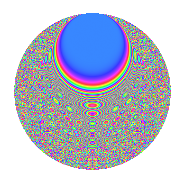# Properties

 Label 8011.2.aLevel 8011 Weight 2 Character orbit a Rep. character $$\chi_{8011}(1,\cdot)$$ Character field $$\Q$$ Dimension 667 Newforms 2 Sturm bound 1335 Trace bound 1

# Related objects

## Defining parameters

 Level: $$N$$ = $$8011$$ Weight: $$k$$ = $$2$$ Character orbit: $$[\chi]$$ = 8011.a (trivial) Character field: $$\Q$$ Newforms: $$2$$ Sturm bound: $$1335$$ Trace bound: $$1$$

## Dimensions

The following table gives the dimensions of various subspaces of $$M_{2}(\Gamma_0(8011))$$.

Total New Old
Modular forms 668 668 0
Cusp forms 667 667 0
Eisenstein series 1 1 0

The following table gives the dimensions of the cuspidal new subspaces with specified eigenvalues for the Atkin-Lehner operators.

$$8011$$Dim.
$$+$$$$309$$
$$-$$$$358$$

## Trace form

 $$667q$$ $$\mathstrut -\mathstrut 4q^{3}$$ $$\mathstrut +\mathstrut 664q^{4}$$ $$\mathstrut +\mathstrut 2q^{5}$$ $$\mathstrut +\mathstrut 6q^{8}$$ $$\mathstrut +\mathstrut 665q^{9}$$ $$\mathstrut +\mathstrut O(q^{10})$$ $$667q$$ $$\mathstrut -\mathstrut 4q^{3}$$ $$\mathstrut +\mathstrut 664q^{4}$$ $$\mathstrut +\mathstrut 2q^{5}$$ $$\mathstrut +\mathstrut 6q^{8}$$ $$\mathstrut +\mathstrut 665q^{9}$$ $$\mathstrut -\mathstrut 2q^{10}$$ $$\mathstrut -\mathstrut 2q^{11}$$ $$\mathstrut -\mathstrut 22q^{12}$$ $$\mathstrut -\mathstrut 4q^{13}$$ $$\mathstrut -\mathstrut 8q^{14}$$ $$\mathstrut -\mathstrut 16q^{15}$$ $$\mathstrut +\mathstrut 654q^{16}$$ $$\mathstrut +\mathstrut 2q^{17}$$ $$\mathstrut +\mathstrut 4q^{18}$$ $$\mathstrut -\mathstrut 14q^{19}$$ $$\mathstrut +\mathstrut 2q^{20}$$ $$\mathstrut +\mathstrut 2q^{21}$$ $$\mathstrut -\mathstrut 14q^{22}$$ $$\mathstrut +\mathstrut 10q^{23}$$ $$\mathstrut +\mathstrut 669q^{25}$$ $$\mathstrut +\mathstrut 8q^{26}$$ $$\mathstrut -\mathstrut 16q^{27}$$ $$\mathstrut +\mathstrut 10q^{28}$$ $$\mathstrut +\mathstrut 28q^{29}$$ $$\mathstrut +\mathstrut 14q^{30}$$ $$\mathstrut -\mathstrut 6q^{31}$$ $$\mathstrut +\mathstrut 22q^{32}$$ $$\mathstrut -\mathstrut 2q^{34}$$ $$\mathstrut -\mathstrut 4q^{35}$$ $$\mathstrut +\mathstrut 664q^{36}$$ $$\mathstrut +\mathstrut 2q^{37}$$ $$\mathstrut -\mathstrut 2q^{39}$$ $$\mathstrut -\mathstrut 12q^{40}$$ $$\mathstrut +\mathstrut 6q^{41}$$ $$\mathstrut -\mathstrut 16q^{42}$$ $$\mathstrut -\mathstrut 18q^{43}$$ $$\mathstrut -\mathstrut 10q^{44}$$ $$\mathstrut +\mathstrut 6q^{45}$$ $$\mathstrut +\mathstrut 10q^{46}$$ $$\mathstrut -\mathstrut 16q^{47}$$ $$\mathstrut -\mathstrut 76q^{48}$$ $$\mathstrut +\mathstrut 665q^{49}$$ $$\mathstrut -\mathstrut 22q^{50}$$ $$\mathstrut -\mathstrut 22q^{51}$$ $$\mathstrut -\mathstrut 22q^{52}$$ $$\mathstrut -\mathstrut 2q^{53}$$ $$\mathstrut -\mathstrut 50q^{54}$$ $$\mathstrut -\mathstrut 20q^{55}$$ $$\mathstrut -\mathstrut 42q^{56}$$ $$\mathstrut -\mathstrut 40q^{57}$$ $$\mathstrut +\mathstrut 44q^{58}$$ $$\mathstrut -\mathstrut 24q^{59}$$ $$\mathstrut -\mathstrut 62q^{60}$$ $$\mathstrut -\mathstrut 10q^{61}$$ $$\mathstrut -\mathstrut 38q^{62}$$ $$\mathstrut -\mathstrut 30q^{63}$$ $$\mathstrut +\mathstrut 650q^{64}$$ $$\mathstrut +\mathstrut 8q^{65}$$ $$\mathstrut -\mathstrut 80q^{66}$$ $$\mathstrut -\mathstrut 24q^{67}$$ $$\mathstrut -\mathstrut 10q^{68}$$ $$\mathstrut +\mathstrut 6q^{69}$$ $$\mathstrut +\mathstrut 12q^{70}$$ $$\mathstrut -\mathstrut 8q^{71}$$ $$\mathstrut +\mathstrut 10q^{72}$$ $$\mathstrut -\mathstrut 12q^{73}$$ $$\mathstrut +\mathstrut 36q^{74}$$ $$\mathstrut -\mathstrut 38q^{75}$$ $$\mathstrut -\mathstrut 58q^{76}$$ $$\mathstrut +\mathstrut 60q^{77}$$ $$\mathstrut -\mathstrut 8q^{78}$$ $$\mathstrut +\mathstrut 2q^{79}$$ $$\mathstrut -\mathstrut 8q^{80}$$ $$\mathstrut +\mathstrut 675q^{81}$$ $$\mathstrut +\mathstrut 50q^{82}$$ $$\mathstrut +\mathstrut 4q^{83}$$ $$\mathstrut +\mathstrut 22q^{84}$$ $$\mathstrut +\mathstrut 6q^{85}$$ $$\mathstrut +\mathstrut 6q^{86}$$ $$\mathstrut +\mathstrut 22q^{87}$$ $$\mathstrut +\mathstrut 14q^{89}$$ $$\mathstrut +\mathstrut 56q^{90}$$ $$\mathstrut -\mathstrut 52q^{91}$$ $$\mathstrut +\mathstrut 46q^{92}$$ $$\mathstrut -\mathstrut 36q^{94}$$ $$\mathstrut +\mathstrut 4q^{95}$$ $$\mathstrut +\mathstrut 44q^{96}$$ $$\mathstrut +\mathstrut 10q^{97}$$ $$\mathstrut +\mathstrut 80q^{98}$$ $$\mathstrut -\mathstrut 2q^{99}$$ $$\mathstrut +\mathstrut O(q^{100})$$

## Decomposition of $$S_{2}^{\mathrm{new}}(\Gamma_0(8011))$$ into irreducible Hecke orbits

Label Dim. $$A$$ Field CM Traces A-L signs $q$-expansion
$$a_2$$ $$a_3$$ $$a_5$$ $$a_7$$ 8011
8011.2.a.a $$309$$ $$63.968$$ None $$-33$$ $$-15$$ $$-74$$ $$-19$$ $$+$$
8011.2.a.b $$358$$ $$63.968$$ None $$33$$ $$11$$ $$76$$ $$19$$ $$-$$